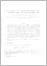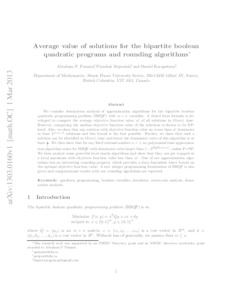# Average value of solutions for the bipartite boolean quadratic programs and rounding algorithms

Punnen, Abraham P and Sripratak, Piyashat and Karapetyan, Daniel (2015) 'Average value of solutions for the bipartite boolean quadratic programs and rounding algorithms.' Theoretical Computer Science, 565. 77 - 89. ISSN 0304-3975Preview
Text
1303.0160v1.pdf - Accepted Version

Download (246kB) | Preview

## Abstract

We consider domination analysis of approximation algorithms for the bipartite boolean quadratic programming problem (BBQP) with m+n variables. A closed-form formula is developed to compute the average objective function value A of all solutions in O(mn) time. However, computing the median objective function value of the solutions is shown to be NP-hard. Also, we show that any solution with objective function value no worse than A dominates at least 2 m+n-2 solutions and this bound is the best possible. Further, we show that such a solution can be identified in O(mn) time and hence the domination ratio of this algorithm is at least 14. We then show that for any fixed natural numbers a and b such that η=ab > 1, no polynomial time approximation algorithm exists for BBQP with domination ratio larger than 1-2(1-η)η(m+n), unless P = NP. It is shown that some powerful local search algorithms can get trapped at a local maximum with objective function value less than A. One of our approximation algorithms has an interesting rounding property which provides a data dependent lower bound on the optimal objective function value. A new integer programming formulation of BBQP is also given and computational results with our rounding algorithms are reported.

Item Type: Article Quadratic programming Boolean variables Heuristics Worst-case analysis Domination analysis Q Science > QA Mathematics Faculty of Science and Health > Computer Science and Electronic Engineering, School of Elements 24 May 2018 10:38 24 May 2018 11:15 http://repository.essex.ac.uk/id/eprint/22057

### Actions (login required)View Item